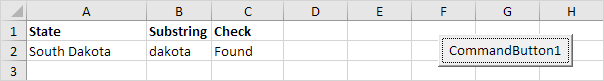# Instr

Use Instr in Excel VBA to find the position of a substring in a string. The Instr function is quite versatile.

Place a command button on your worksheet and add the code lines below. To execute the code lines, click the command button on the sheet.

### Simple Instr Function

By default, the Instr function starts searching at the beginning of the string (position 1).

Code:

Dim state As String

state = “Virginia”

MsgBox InStr(state, “gin”)

Result: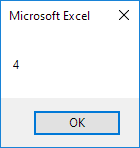Note: string “gin” found at position 4.

### Start Position

The second Instr function below starts searching at position 7.

Code:

Dim state As String

state = “South Carolina”

MsgBox InStr(state, “o”)

MsgBox InStr(7, state, “o”)

Result: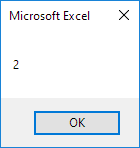Explanation: the first Instr function finds the string “o” at position 2. The second Instr function starts searching at position 7 and finds the string “o” at position 10.

### Zero

The Instr function returns 0 if the string is not found (important as we will see next).

Code:

Dim state As String

state = “Florida”

MsgBox InStr(state, “us”)

Result:### Instr and If

Let’s create a simple VBA program that uses the Instr function.

Code:

Dim state As String, substring As String

state = Range(“A2”).Value

substring = Range(“B2”).Value

If InStr(state, substring) > 0 Then

Range(“C2”).Value = “Found”

Else

End If

Result when you click the command button on the sheet: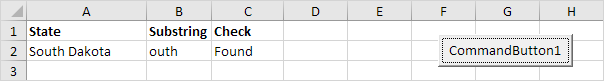Explanation: string “outh” found at position 2. The Instr function returns 2. As a result, Excel VBA places the string “Found” into cell C2.

### Case-insensitive Search

By default, the Instr function performs a case-sensitive search. Enter the string “dakota” into cell B2 and click the command button on the sheet.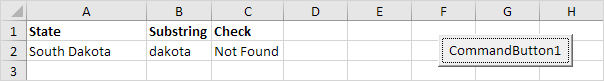Explanation: string “dakota” not found (first letter not capitalized). The Instr function returns 0. As a result, Excel VBA places the string “Not Found” into cell C2.

To perform a case-insensitive search, update the code as follows:

Dim state As String, substring As String

state = Range(“A2”).Value

substring = Range(“B2”).Value

If InStr(1, state, substring, vbTextCompare) > 0 Then

Range(“C2”).Value = “Found”

Else